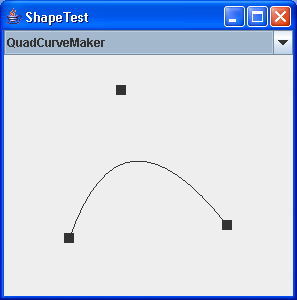# fillRect (int, int, int, int) method draws a solid rectangle : Shape « 2D Graphics GUI « Java

fillRect (int, int, int, int) method draws a solid rectangle

```
import java.awt.Graphics;

import javax.swing.JFrame;
import javax.swing.JPanel;

public class MainClass extends JPanel {

public static void main(String[] a) {
JFrame f = new JFrame();
f.setSize(400, 400);
f.setDefaultCloseOperation(JFrame.EXIT_ON_CLOSE);
f.setVisible(true);
}

public void paint(Graphics g) {
g.fillRect (5, 15, 50, 75);
}
}

```

### Related examples in the same category

 1 Creating Basic Shapes 2 Creating a Shape Using Lines and Curves 3 Combining Shapes 4 Draw rectangles, use the drawRect() method. To fill rectangles, use the fillRect() method 5 Draw line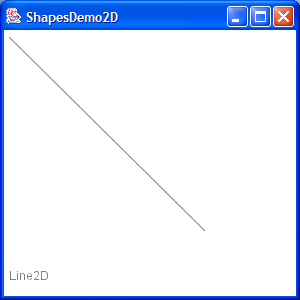6 Draw a Polygon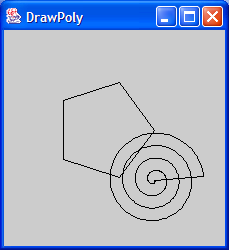7 Draw an oval outline 8 Draw a (Round)rectangle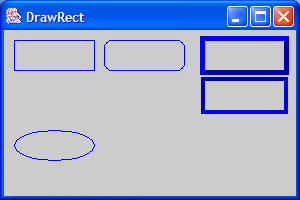9 Fill a polygon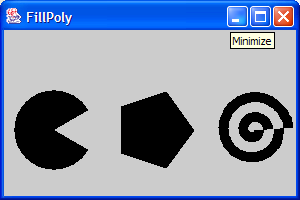10 Fill a solid oval 11 Fill a (Round)rectangle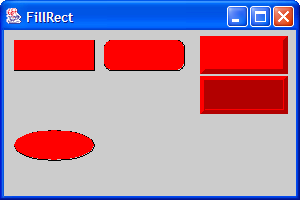12 Change font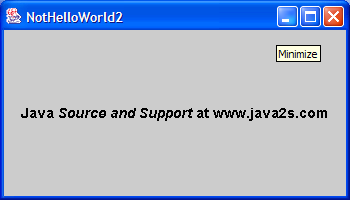13 Draw rectangle 2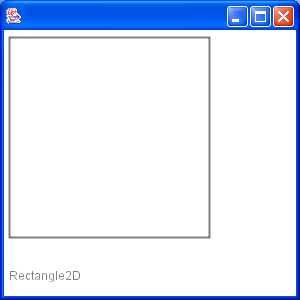14 Draw Arc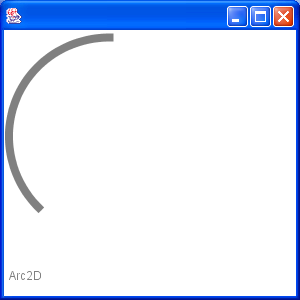15 Draw Ellipse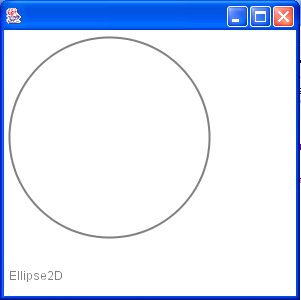16 Fill a Rectangle 2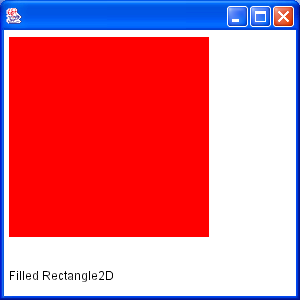17 Fill Arc 2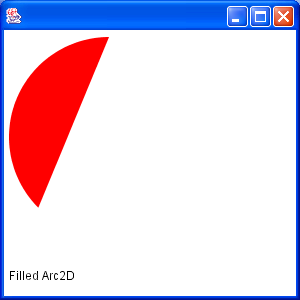18 Draw text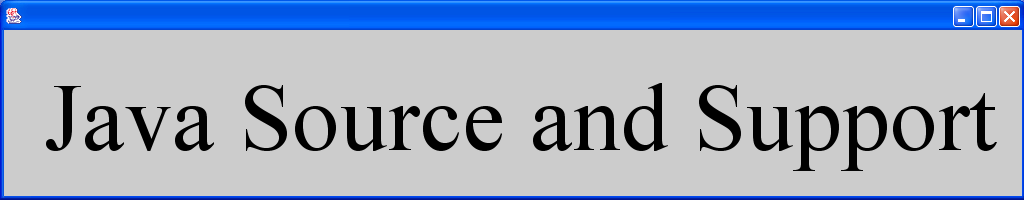19 Draw unicode string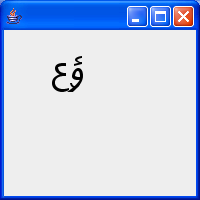20 Shape combine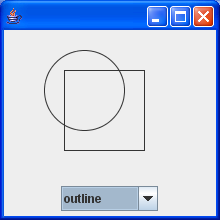21 Effects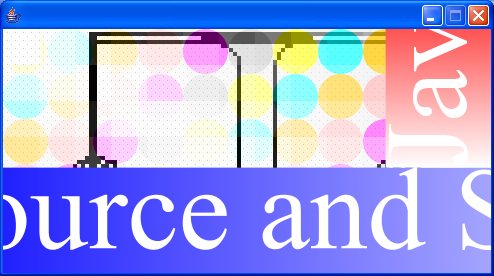22 Mouse drag and drop to draw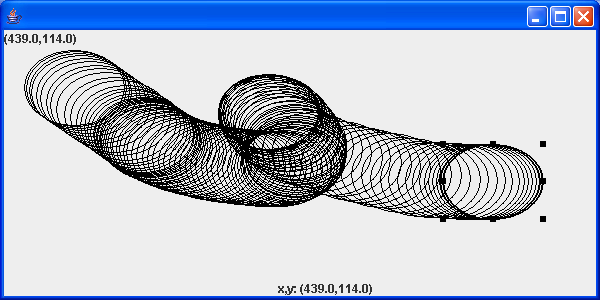23 Arc demonstration: scale, move, rotate, sheer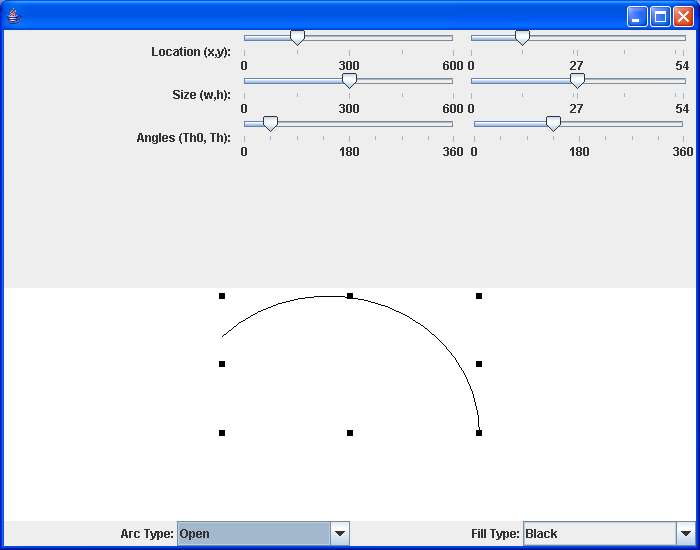24 Hypnosis Spiral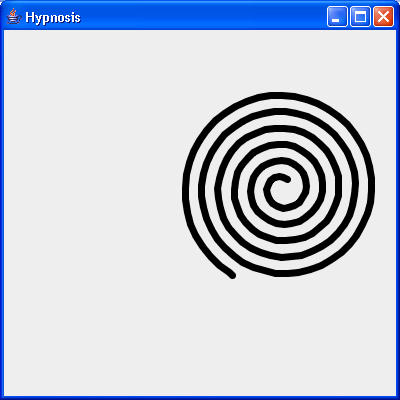25 GlyphVector.getNumGlyphs() 26 Resize a shape 27 Rectangle with rounded corners drawn using Java 2D Graphics API 28 Compares two ellipses and returns true if they are equal or both null. 29 Compares two lines are returns true if they are equal or both null. 30 Creates a diagonal cross shape. 31 Creates a diamond shape. 32 Creates a new Stroke-Object for the given type and with. 33 Creates a region surrounding a line segment by 'widening' the line segment. 34 Creates a triangle shape that points downwards. 35 Creates a triangle shape that points upwards. 36 Generate Polygon 37 Polygon with float coordinates. 38 Polyline 2D 39 Serialises a Shape object. 40 Tests two polygons for equality. If both are null this method returns true. 41 Union two rectangles 42 Calculate Intersection Clip 43 Draws a shape with the specified rotation about (x, y). 44 Checks, whether the given rectangle1 fully contains rectangle 2 (even if rectangle 2 has a height or width of zero!). 45 Reads a Point2D object that has been serialised by the writePoint2D(Point2D, ObjectOutputStream)} method. 46 Returns a point based on (x, y) but constrained to be within the bounds of a given rectangle. 47 RectListManager is a class to manage a list of rectangular regions. 48 Fill Rectangle2D.Double and Ellipse2D.Double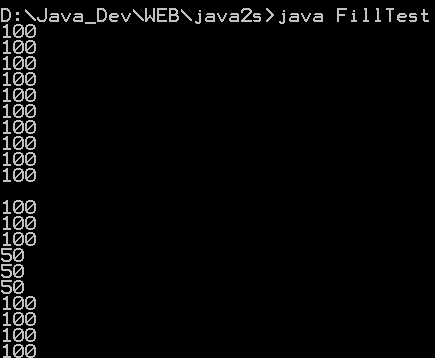49 This program demonstrates the various 2D shapes